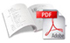Geographia Technica, Vol 14, Issue no.2/2019, pp. 97-111

# Andrii SRYBERKO (Odesa, Ukraine)

DOI: 10.21163/GT_2019.142.09

ABSTRACT: The studies on calculation of vertical distribution of water temperature in the Black Sea by satellite data are described. The research was carried out in the active layer of the deep part of the Black Sea at depths of 0 – 50 meters. The initial data of the actual water temperature values were station data or measurement from the ship data (OSD – Ocean Station data), data measured with the help of floats (PFL – Profiling float data) and satellite data of the sea surface temperature. Calculations were based on the definition of statistical dependencies between the values of water temperature at the neighboring levels of vertical temperature distribution in the Black Sea by OSD & PFL data of water temperature and the development of regression equations. Calculations showed statistically significant results in spring-autumn period. Correlation coefficients between the values of the water temperature on the neighboring levels in the Black Sea amounted to 0.88 – 0.99. To increase the accuracy of results the equations for the calculation of adjustments for the temperature of the water at depths: 10, 20, 25 and 50 meters were developed. Standard calculation errors of the vertical distribution of water temperature amounted to 2 °C in the Black Sea in 2017 year.

Keywords: Black Sea, water temperature calculation, correlation coefficient, statistical dependence, regression equation, satellite data.Full article here